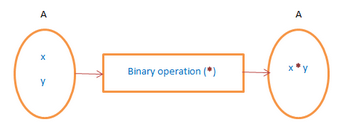Courses

# Binary Operations Mathematics Notes | EduRev

## Algebra for IIT JAM Mathematics

Created by: Veda Institute

## Mathematics : Binary Operations Mathematics Notes | EduRev

The document Binary Operations Mathematics Notes | EduRev is a part of the Mathematics Course Algebra for IIT JAM Mathematics.
All you need of Mathematics at this link: Mathematics

We are quite familiar with arithmetic operations like addition, subtraction, division, and multiplication. Also, we know about exponential function, log function etc. Today we will learn about the binary operations. As the name suggests, binary stands for two. Does that mean that we can use two functions simultaneously using binary operation? Let’s find out.

Binary Operation
Just as we get a number when two numbers are either added or subtracted or multiplied or are divided. The binary operations associate any two elements of a set. The resultant of the two are in the same set. Binary operations on a set are calculations that combine two elements of the set (called operands) to produce another element of the same set.
The binary operations * on a non-empty set A are functions from A × A to A. The binary operation, *: A × A → A. It is an operation of two elements of the set whose domains and co-domain are in the same set.Addition, subtraction, multiplication, division, exponential is some of the binary operations.

Properties of Binary Operation

• Closure property: An operation * on a non-empty set A has closure property, if a ∈ A, b ∈ A ⇒ a * b ∈ A.
• Additions are the binary operations on each of the sets of Natural numbers (N), Integer (Z), Rational numbers (Q), Real Numbers(R), Complex number(C).

The additions on the set of all irrational numbers are not the binary operations.

• Multiplication is a binary operation on each of the sets of Natural numbers (N), Integer (Z), Rational numbers (Q), Real Numbers(R), Complex number(C).

Multiplication on the set of all irrational numbers is not a binary operation.

• Subtraction is a binary operation on each of the sets of Integer (Z), Rational numbers (Q), Real Numbers(R), Complex number(C).

Subtraction is not a binary operation on the set of Natural numbers (N).

• A division is not a binary operation on the set of Natural numbers (N), integer (Z), Rational numbers (Q), Real Numbers(R), Complex number(C).
• Exponential operation (x, y) → xy is a binary operation on the set of Natural numbers (N) and not on the set of Integers (Z).

Types of Binary Operations

Commutative
A binary operation * on a set A is commutative if a * b = b * a, for all (a, b) ∈ A (non-empty set). Let addition be the operating binary operation for a = 8 and b = 9, a + b = 17 = b + a.

Associative
The associative property of binary operations hold if, for a non-empty set A, we can write (a * b) *c = a*(b * c). Suppose N be the set of natural numbers and multiplication be the binary operation. Let a = 4, b = 5 c = 6. We can write (a × b) × c = 120 = a × (b × c).

Distributive
Let * and o be two binary operations defined on a non-empty set A. The binary operations are distributive if a*(b o c) = (a * b) o (a * c) or (b o c)*a = (b * a) o (c * a). Consider * to be multiplication and o be subtraction. And a = 2, b = 5, c = 4. Then, a*(b o c) = a × (b − c) = 2 × (5 − 4) = 2. And (a * b) o (a * c) =  (a × b) − (a × c) = (2 × 5) − (2 × 4) = 10 − 6 = 2.

Identity
If A be the non-empty set and * be the binary operation on A. An element e is the identity element of a ∈ A, if a * e = a = e * a. If the binary operation is addition(+), e = 0 and for * is multiplication(×), e = 1.

Inverse
If a binary operation * on a set A which satisfies a * b = b * a = e, for all a, b ∈ A. a-1 is invertible if for a * b = b * a= e, a-1 = b. 1 is invertible when * is multiplication.

Solved Example for You

Problem: Show that division is not a binary operation in N nor subtraction in N.

Solution: Let a, b ∈ N
Case 1: Binary operation * = division(÷)
: N × N→N given by (a, b) (a/b) ∉ N (as 5/3 ∉ N)
Case 2: Binary operation * = Subtraction(−)
: N × N→N given by (a, b) a − b ∉ N (as 3 − 2 = 1 ∈ N but 2−3 = −1 ∉ N).

Offer running on EduRev: Apply code STAYHOME200 to get INR 200 off on our premium plan EduRev Infinity!

,

,

,

,

,

,

,

,

,

,

,

,

,

,

,

,

,

,

,

,

,

;